# Concept of analytical reasoning, Types of analytical reasoning questions and Important pointsPosted on : 25-12-2018 Posted by : Admin## Concept of analytical reasoning

Analytical reasoning involves questions where the candidates need to count the number of geometrical shapes present in the given complex question figure. These types of questions require a systematic approach. First of all candidate must analyze the given complex question figure and then go on to determine the number of shapes in question.

These types of questions are very important in competitive exams like GRE, Bank exams, Civil services, IBPS PO and Clerk. So let us study well and score more on this topic.

A few examples of the types of questions asked in the exam are given below,

## Types of analytical reasoning questions

There are many types of embedded figure questions. A few of them are explained below,

### Type 1: Counting number of straight lines

Consider the following example,

In each of the following question, find the minimum number of straight lines required to make the given figure.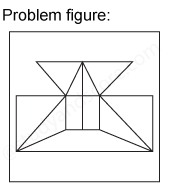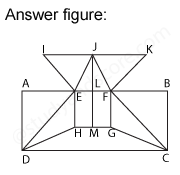After labelling the figure, we get the following,

• The horizontal lines are AD, EH, JM, FG, and BC (5)
• The slanting lines are IE, JE, JF, KF, DE,DH,FC and GC (8)

Total number of straight lines in the figure are 4+5+8=17

### Type 2: Counting number of triangles

Consider the following example,

In each of the following question, find the minimum number of triangles in the given figure.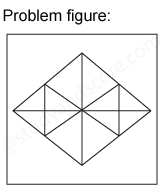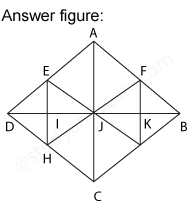After labelling the figure, we get the following,

• Simplest triangles AFJ, FJK, FKB, BKG, JKG, JGC, HJC, HIJ, DIH, DEI, EIJ and AEJ (12)
• Triangles with two components each JFB, FBG, BJG, JFG, DEJ, EJH, DJH and DEH (8)
• Triangles with three components each AJB, JBC, DJC and ADJ (4)
• Triangles with six components each DAB, ABC, BCD and ADC (4)

Total number of triangles in the figure is 12 + 8 + 4 + 4 = 28

### Type 3: Counting the number of squares

Consider the following example,

In each of the following questions, count the number of squares in the figure.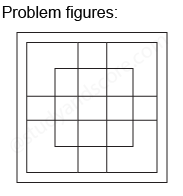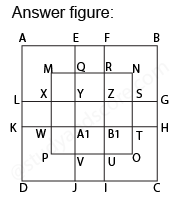After labelling the figure, we get the following,

• Simplest squares are EFRQ, MQYX, QRZY, RNSZ, LXWK, XYA, W, YZB1 A1, ZSTB1, SGHT, WA1VP, A1B1UV, B1TOPU and VUIJ (13)
• Squares with two components each AEYL, FBGZ, KA1JD and B1HCI (4)
• Squares with four components each MRB1W, QNTA1, XZUP and YSOV (4)
• Squares with seven components each AFB1K, EBHA1, LZID and YGCJ (4)
• Square with nine components is MNOP (1)
• Square with seventeen components is ABCD (1)

Total number of squares in the figure is 13 + 4 + 4 + 4 + 1 + 1 = 27

### Type 4: Counting number of parallelograms

Consider the following example,

In each of the following question, count the number of parallelogram in the given figure.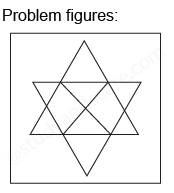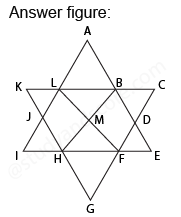After labelling the figure, we get the following,

• Simplest parallelograms LMHJ and BDFM (2) Parallelograms with two components each ABML and MFGH (2)
• Prallelograms with three components each LBHI, LBEF, BDGH, DFLA, BCFH, KLFH, ABHJ and LFGJ (8)
• Parallelogram composed of six components is LCFI, KBEH and ADGJ (3)

Total number of parallelograms in the figure is 2 + 2 + 8 + 3 = 15

### Type 5: Counting number of rectangles

How many rectangles are there in the following figure.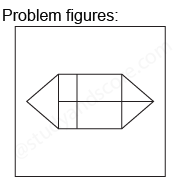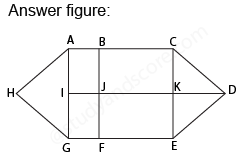After labelling the figure, we get the following,

• Simplest rectangles ABBJI, BCKJ, IJFG, and JKEF (4)
• Rectangles with two components each ACKI, BCEF, IKEG, and ABFG (4)
• Rectangles composed of four components is ACEG (1)

Total number of Rectangles in the figure is 4 + 4 + 1 = 9

### Type 6: Counting two different shapes

In each of the the following question, count the number of triangles and squares in the given figure.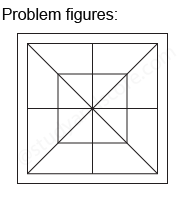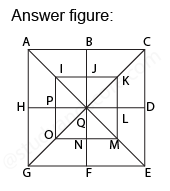After labelling the figure, we get the following, Simplest triangles IJQ, JKQ, KLQ, LMQ, MNQ, NOQ, OPQ, and PIQ (8)

• Triangles with two components each ABQ, BCQ, CDQ, DEQ, EFQ, FGQ, GHQ, HAQ, IKQ, KMQ, MOQ, and OIQ (12)
• Triangles with four components each ACQ, CEQ, EGQ, GAQ, IKM, KMO, MOI and OIK (8)
• Triangles with eight components each ACE, CEG, EGA and GAC (4)

Total number of triangles in the figure is 8 + 12 + 8 + 4 = 32

Also we need to count the number of squares in this figure,

• Squares with two components each IJQP, JKLQ, QLMN, and PQNO (4)
• Squares with four components each ABQH, BCDQ, QDEF and HQFG (4)
• Square with eight components is IKMO (1)
• Square with sixteen components is ACEG (1)

Total number of squares in the figure is 4 + 4 + 1 + 1 = 10

Hence option C is correct.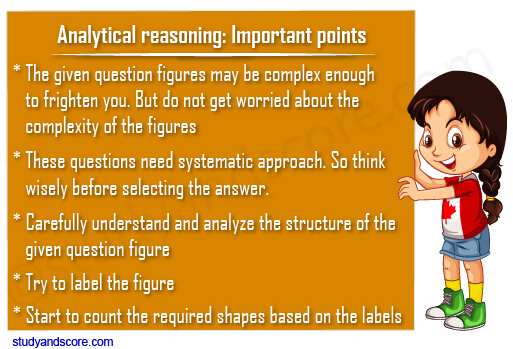Hope you have liked this post.